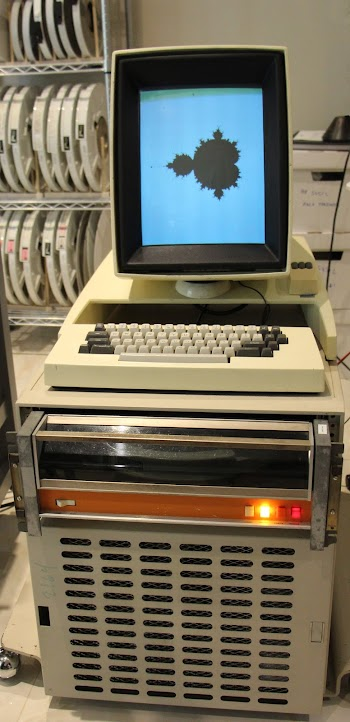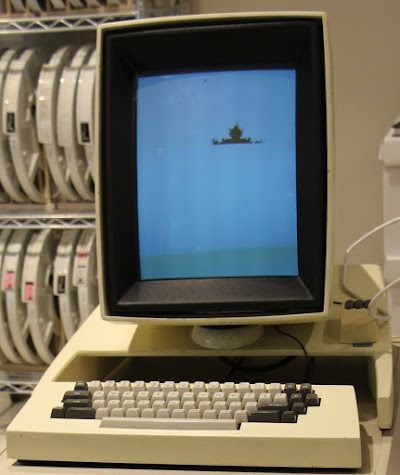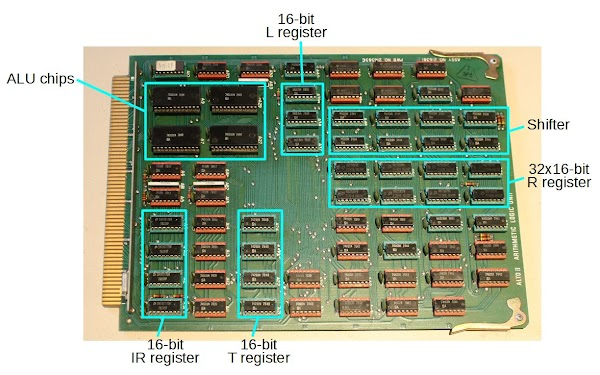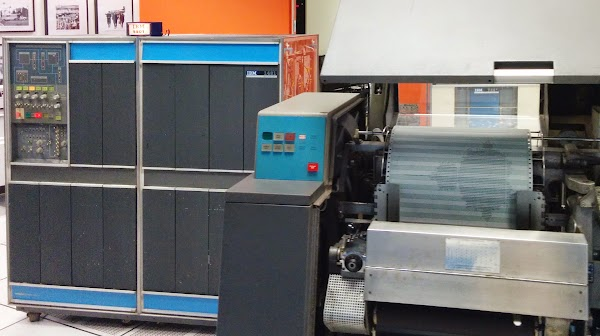### One-hour Mandelbrot: Creating a fractal on the vintage Xerox Alto

I wrote a short program to generate the Mandelbrot set on the Xerox Alto, a groundbreaking minicomputer from the 1970s. The program, in the obsolete BCPL language, ran very slowly—taking almost exactly an hour—but the result below shows off the Alto's monochrome bitmapped display. (Bitmapped displays were a rarity at the time because memory was so expensive.)The Xerox Alto took an hour to generate the Mandelbrot set.

The Alto was a revolutionary computer designed at Xerox PARC in 1973 to investigate personal computing. It introduced the GUI, Ethernet and laser printers to the world, among other things. In the photo above, the Alto computer itself is in the lower cabinet. The Diablo disk drive (with the 1970s orange stripe) uses a removable 14 inch disk pack that stores 2.5 megabytes of data. (A bunch of disk packs are visible behind the Alto.) The Alto's display is bitmapped with 606x808 pixels in an unusual portrait orientation, and the optical mouse is next to the display.

Last year Y Combinator received an Alto from computer visionary Alan Kay and I'm helping restore the system, along with Marc Verdiell, Luca Severini and Carl Claunch. My full set of Alto posts is here and Marc's videos are here. I haven't posted an update for a while, but now I can write new programs and download them to the Alto using the Living Computer Museum's Alto file system implementation and gateway to the Alto's 3Mb Ethernet. I decided to start with the Mandelbrot set to take advantage of the Alto's high resolution display.

Marc's latest video shows the Mandelbrot programming running on the Alto.

## The Mandelbrot program

The Mandelbrot set algorithm is fairly simple. Each point on the plane represents a complex number c. You repeatedly iterate the complex function f(z) = z^2 + c. If the value diverges to infinity, the point is outside the set. Otherwise, the point is inside the set and the pixel is set to black. Setting the pixel is tricky because the Alto doesn't have a graphics API; you need to determine which bit in memory to set.4

Since the Xerox Alto doesn't support floating point1, I needed a way to represent the numbers with its 16-bit word. I use fixed point arithmetic: 4 bits to the left of the decimal point and 12 bits to the right.2 For instance, the number 1.25 is represented in 16 bits as 1.25*2^12 = 0x1400. These fixed point numbers can be added with standard integer addition. After multiplying two fixed point numbers with integer multiplication, the 32-bit result must be divided by 2^12 (i.e. shifted right by 12) to restore the decimal point location.3

The code (above) is written in BCPL, the main language used on the Alto. BCPL is a precursor to C and many features of C are clearly visible in BCPL: everything from lvalues and rvalues to the ternary operator. You can think of BCPL as C without types; the only BCPL types are 16-bit words along with C-like structs, unions and bitfields. BCPL may look unfamiliar at first, but the code above should be clear if you consider the following syntax differences with C:

• Blocks are indicated with [ and ] instead of { and }.
• Indexing is with a!1 instead of a.
• And, Or, and Shift bit operations are &, %, and lshift/rshift.
• Variable definitions use let.
• Arrays are defined with vec.

More information on BCPL is in the BCPL Reference Manual and my earlier article on using BCPL with the Alto.The Xerox Alto, a few minutes into generation of the Mandelbrot set.

## Why is the Alto so slow?

Running the Mandelbrot set illustrates the amazing improvement in computer speed since the Alto was created in 1973 and the huge changes in computer architecture. On a modern computer, a Javascript program can generate the Mandelbrot set in a fraction of a second, while the Alto took an hour. The first factor is the Alto's slow clock speed of 5.88 MHz, hundreds of times slower than a modern processor. In addition, the Alto doesn't execute machine instructions directly, but uses a relatively inefficient microcode emulator that takes many cycles to perform one machine instruction.The ALU board from the Xerox Alto. The Alto doesn't use a microprocessor chip, but a CPU built from three boards of integrated circuits.

Unlike modern computers, the Alto doesn't use a microprocessor chip, but instead has a CPU built from three boards full of simple TTL chips. The photo above shows the arithmetic-logic unit (ALU) board, which uses four 4-bit 74181 ALU chips to perform addition, subtraction and logic operations. You can also see the CPU's registers on this board. The Alto doesn't include a hardware multiplier, but must perform multiplication by repeated shifts and adds. Thus, the Alto performs especially poorly on the Mandelbrot set, which is essentially repeated multiplications.

## Conclusion

The Mandelbrot set was a quick program to try out the Alto's graphics. Next I'll try some more complex projects on the Alto. If you want to run my code, it's on Github; you can run it on the ContrAlto simulator if you don't have an Alto available.

If you're interested in retrocomputing fractals, I also generated a Mandelbrot on a 50 year old IBM 1401 mainframe The 1401 generated the Mandelbrot set in 12 minutes—not because it's a faster machine than the Alto, but because the resolution on the line printer was very very low.Mandelbrot generated on the IBM 1401 mainframe.

## Notes and references

1. There is a floating point library (source) for the Alto. I decided not use use it since the integer Mandelbrot was already very slow. But using floating point would make sense if you wanted to zoom in on the Mandelbrot.

2. Fixed-point arithmetic is a common trick for fast Mandelbrot calculation.

3. To multiply two 16-bit numbers efficiently, I use the double precision MulFull function (written in Nova assembler) in PressML.asm, part of the Computer History Museum's archived Alto software.

4. The hardest part of generating the Alto Mandelbrot was figuring out how to configure the display memory and update it correctly. The details on how the display works are in chapter 4 of the Xerox Alto Hardware Manual. To summarize, the display contents are defined by a linked list of display control blocks (DCBs), which define a rectangular region of pixels on the display. A microcode task reads 16 words of pixels from memory at a time and writes them to the display board, which shifts the pixels out to the monitor. Thus, as each scanline is being written to the CRT, the CPU is busily reading the pixels for that line from memory and feeding them to the display, another reason why the Alto is slow.

The Alto's Smalltalk environment has a simple graphics API, but we don't have Smalltalk running yet.

Unknown said...

I think the Alto had languages that made much better use of the hardware than compiling to Data General Nova instructions and then emulating the Nova with microcode. The Nova stuff was just there for bootstrapping before PARC had time to write better software and microcode. So if you were interested in reducing the time to compute the Mandelbrot set you might try Cedar or something.

Of course just getting the machine to power on is a tour de force.

Paul McJones said...

@David, the Alto indeed had other languages, as well as a writable control store, so a language environment or even an application could load custom microcode. But "Novacode" was widely used, e.g.: Bravo, Markup, Draw, the Interim File Server, etc. The Cedar system, with its Cedar Mesa programming language, ran on the much faster Dorado rather than the Alto.

Unknown said...

Thanks for another post. I saw Marc was still posting on youtube, so I was wondering if you would keep blogging about it. The Mandelbrot set was a great idea to showcase the bit mapping capabilities of the alto, which were definitely ahead of it's time. I don't know about faster programming languages for the Alto, but you could probably greatly decrease the time required by disabling the screen redrawing subroutine (if possible) until the program is finished.

Paul McJones said...

@Lord, the screen redrawing is done in microcode, and you are correct that it slows things down -- by about a factor of three (see http://bwlampson.site/38-AltoSoftware/ThackerAltoHardware.pdf). It could be shut off during the computation phase by moving the statement "lvdas!0 = dcb" down to the end.

Snial said...

If I understand the Nova instruction set properly, lshift n and rshift n must be performed by multiple single bit shifts and the Alto implementation of the BCPL (aka Nova) instruction set doesn't extend it with multiple bit shifts.

This implies that the code for lshift n and rshift n is either unwound into 16 shifts in total, which is fairly slow or implemented as a call to a library routine involving a loop which would be even slower.

So, replacing the lshift and rshift n by a division could be faster if we use microcoded single precision div:

{iiii:ffffffffffff} * {iiii:ffffffffffff} => {IIIIIIII:FFFFFFFF}:{FFFFFFFFFFFFFFFF} / 4096.

The standard BCPL division is signed, however, PressML actually includes a MulDiv operation which performs unsigned multiply then divide using the microcode instructions with an intermediate 32-bit value. This should work if we manually adjust for signed arithmetic. Thus we can do:

let x2sp =(x ls 0) ? -x,x // x^2 will be +ve.
x2sp = MulDiv(x2sp,x2sp,4096) // yield x^2 single precision.
let y2sp = (y ls 0) ? -y,y // y^2 will be +ve
y2sp = MulDiv(y,y,4096) // yield = y^2, single precision
if x2sp+ y2sp ge 16384 then break // Quit if x^2 + y^2 > 4

in place of:

MulFull(y, y, y2) // y2 = y*y. Integer multiplication, y2 is double word.
MulFull(x, x, x2) // x2 = x*x
if x2!0 + y2!0 ge 1024 then break // Quit if x^2 + y^2 > 4

if n eq 20 then // Last iteration. Still inside set, so set pixel.
[
let adr = (200 + ypos) * 38 + h // 200 blank pixels at top, 38 words per line
break
]

// Convert to single precision by dropping 12 bits.
// rshift 12 = lshift top word 4
let x2sp = (x2!0 lshift 4) % (x2!1 rshift 12) // x2sp = x^2, single precision
let y2sp = (y2!0 lshift 4) % (y2!1 rshift 12) // y2sp = y^2, single precision

MulFull(x, y, xy) // xy = x*y
let xysp = (xy!0 lshift 4) % (xy!1 rshift 12) // xysp = x*y, single precision

we would have:

let xysgn=x xor y // top bit is sign for result.
if x<0 then x=-x
if y<0 then y=-y
x=MulDiv(x,y,4096) // the unsigned version.
if xysgn<0 then x=-x // correct the sign.

y=x + x + cy
x=x2sp - y2sp + cx

In addition, the double checking of the exit conditions surely makes things slower. I would do:

n=20
[
// ... as before
n=n-1 // dsz, nop.
]repeatuntil n ls 0

The display update code is still fairly slow owing to the multiply and the shift:

if n ls 0 then // Still inside set, so set pixel.
[
let adr = (200 + ypos) * 38 + h // 200 blank pixels at top, 38 words per line
break
]

We don't use v again, so I'd set it to the right address at the outer loop:

v=v+200*38 // start 200 scans down.
let cy=y0...

I'd increment it after the end of the b loop:
for b = 0 to 15 do // horizontal bit count
[
... as before.
]
v=v+1 // isz nop.

Since it's addressed linearly, this takes care of both the *38 and +h.

Lastly, I'd use a simple bitmask for handling the pixel, and because the only use for b is the loop counter it makes that part of the code including the end of the n loop:

let b=32768 // horizontal bit mask
[
n=20
[
// ... as before
n=n-1 // dsz, nop.
]repeatuntil n ls 0
if n ls 0 then v!0=v!0 % b // set the pixel
b=b rshift 1
]repeatwhile b
v=v+1

-cheers from Julz

Snial said...

get "streams.d"
external
[
Ws;
Wns;
MulFull;
keys;
Gets;
]

let Main() be
[
let v = vec 30705 // Pixel buffer
v = (v + 1) & -2 // Data needs to be 32-bit aligned
let dcb = vec 5 // Display control block: defines display region
dcb = (dcb + 1) & -2
dcb!0 = 0 // End of display list
dcb!1 = 38 // 38 words per line
dcb!2 = v // Data pointer
dcb!3 = 404 // # lines / 2
let lvdas = #420 // Address holds pointer to display control block
lvdas!0 = dcb

for i = 0 to 30703 do v!i = 0 // Clear display

// Values are represented as fixed point with 12 bits to right of decimal point.
let x0 = (-2) lshift 12 // Left boundary: x = -2
let x1 = 1 lshift 12 // Right boundary: x = 1
let y0 = (-1) lshift 12 // Top boundary: y = -1
let y1 = 1 lshift 12 // Bottom boundary: y = 1
let xstep = (x1 - x0) / 600 // Render 600 pixels horizontally
let ystep = (y1 - y0) / 400 // Render 400 pixels vertically
let x2 = vec 2 // double word to hold x^2
let y2 = vec 2 // double word to hold y^2
let xy = vec 2 // double word to hold x * y

v=v+200*38 // start 200 scans down.
let cy = y0 // Constant value, y part. I.e. the z value for this pixel
for ypos = 0 to 400 do // line count. Note "for" limits are inclusive.
[
let cx = x0 // Constant value, x part.
for h = 0 to 37 do // horizontal word count
[
let b = 32768 // horizontal bit count
[
let x = cx // The complex z value is represented as x + i*y
let y = cy
let n=20
[
let x2sp =(x ls 0) ? -x,x // x^2 will be +ve anyway, so just abs.
x2sp = MulDiv(x2sp,x2sp,4096) // yield x^2 single precision.
let y2sp = (y ls 0) ? -y,y // ditto for y^2.
y2sp = MulDiv(y,y,4096) // yield = y^2, single precision
if x2sp+ y2sp ge 16384 then break // Quit if x^2 + y^2 > 4

let xysgn=x xor y // top bit is sign for result.
if x ls 0 then x=-x
if y ls 0 then y=-y
x=MulDiv(x,y,4096) // the unsigned version.
if xysgn ls 0 then x=-x // correct the sign.

// z = z^2 + c (complex arithmetic, z = x+iy)
// i.e. y = 2xy + cy
// x = x^2 - y2 + cx
y=x + x + cy
x=x2sp - y2sp + cx
n=n-1
]repeatuntil n eq 0

if n eq 0 then v!0=v!0 % b // set the pixel
cx = cx + xstep // Move to next cx value
b=b rshift 1 // next bitmask (rshift is unsigned)
]repeatwhile b // until all the bits in this video word are done.
v=v+1 // next video word.
]
cy = cy + ystep // Move to next cy value
]
Gets(keys) // Get a key, i.e. wait for a keypress
]

Snial said...

Finally, apologies, the external[..] entry for MulFull should be to MulDiv

-best regards from Julz

Paul McJones said...

@Snial/Julz, you say "If I understand the Nova instruction set properly, lshift n and rshift n must be performed by multiple single bit shifts and the Alto implementation of the BCPL (aka Nova) instruction set doesn't extend it with multiple bit shifts." Actually, the Alto's standard microcode includes some augmented instructions, described starting on page 16 of the hardware manual (http://www.bitsavers.org/pdf/xerox/alto/Alto_Hardware_Manual_Aug76.pdf). One of these is:

CYCLE (60000B):
Left cycle (rotate) the contents of ACO by the amount specified in instruction bits 12-15, unless this value is zero, in which case cycle ACO left by the amount specified in ACI. Leaves ACI = cycle count mod 20B.

Snial said...

Hello Paul,

Thanks for that, I should have checked properly. Optimising Mandelbrot sets are fun. In the late 1980s I had a 68008 based Sinclair QL and wrote an assembler version which would display a Mandelbrot set on its 256x256 x 8 colour mode, in 8 colours. It was possible to get it down to about 2 minutes 40s and I think I had the equivalent of a larger value of n. I could also zoom in on quadrants, but the fixed-point limitations did show up before long ;-)

Snial said...

Hello Paul,

Although the Alto itself supports microcode rotates, that's not the same as a shift. Doesn't this file imply, the BCPL compiler makes a call to library routines for shifts?

http://xeroxalto.computerhistory.org/Indigo/AltoSource/BCPLSOURCES.DM!1_/.BUTIL.asm.html

And it can't use those routines to optimise a shift by a constant into a jump into the unrolled loop, because it hasn't set up FRET.

Thus, this brings us back to my original comment: shifts will be slow.

Rotates, I think can be converted into shifts, by using the cycle function a second time to generate a mask. Thus, a shift left of n can be done as:

dest = (src rol n) & ~((1 rol n)-1)

A shift right (assuming rol isn't a rotate through carry) is:

dest = (src rol (16-n)) & ((1 rol (16-n)-1)

It's not clear to me that this would be any faster on average on an Alto. The average time (with the display on) for 16 shifts will be in order of 2.5us*3 = 7.5us per instruction *36 nova instructions = 270us per rounding operation. That's about 1ms for all 3 giving at least 20ms per black pixel.

Paul McJones said...

@Snial, then there is this BCPL microcode: http://xeroxalto.computerhistory.org/Indigo/AltoSource//BCPLRUNTIMESOURCE.DM!1_/.BcplUtil.mu.html

; Right shift
; Computes ac0 ← ac0 rshift ac1
; Called by jsr @347
; Note that shift count may be either positive or negative

...

Ken Shirriff said...

Thanks for all the suggestions for improving performance. I tried them out and Mandelbrot runtime is now 9 minutes instead of an hour. Details are in my new post.